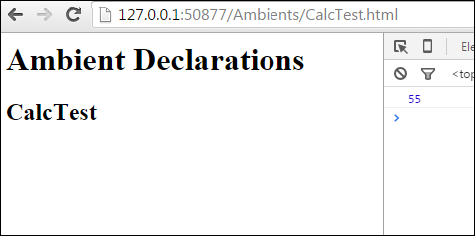# TypeScript - Ambients

Ambient declarations are a way of telling the TypeScript compiler that the actual source code exists elsewhere. When you are consuming a bunch of third party js libraries like jquery/angularjs/nodejs you can’t rewrite it in TypeScript. Ensuring typesafety and intellisense while using these libraries will be challenging for a TypeScript programmer. Ambient declarations help to seamlessly integrate other js libraries into TypeScript.

## Defining Ambients

Ambient declarations are by convention kept in a type declaration file with following extension (d.ts)

```Sample.d.ts
```

The above file will not be transcompiled to JavaScript. It will be used for type safety and intellisense.

The syntax for declaring ambient variables or modules will be as following −

### Syntax

```declare module Module_Name {
}
```

The ambient files should be referenced in the client TypeScript file as shown −

```/// <reference path = " Sample.d.ts" />
```

### Example

Let’s understand this with help of an example. Assume you been given a third party javascript library which contains code similar to this.

```FileName: CalcThirdPartyJsLib.js
var TutorialPoint;
(function (TutorialPoint) {
var Calc = (function () {
function Calc() {
}
Calc.prototype.doSum = function (limit) {
var sum = 0;

for (var i = 0; i <= limit; i++) {
Calc.prototype.doSum = function (limit) {
var sum = 0;

for (var i = 0; i <= limit; i++) {
sum = sum + i;
return sum;
return Calc;
TutorialPoint.Calc = Calc;
})(TutorialPoint || (TutorialPoint = {}));
var test = new TutorialPoint.Calc();
}
}
}
}
}
```

As a typescript programmer you will not have time to rewrite this library to typescript. But still you need to use the doSum() method with type safety. What you could do is ambient declaration file. Let us create an ambient declaration file Calc.d.ts

```FileName: Calc.d.ts
declare module TutorialPoint {
export class Calc {
doSum(limit:number) : number;
}
}
```

Ambient files will not contain the implementations, it is just type declarations. Declarations now need to be included in the typescript file as follows.

```FileName : CalcTest.ts
/// <reference path = "Calc.d.ts" />
var obj = new TutorialPoint.Calc();
obj.doSum("Hello"); // compiler error
console.log(obj.doSum(10));
```

The following line of code will show a compiler error. This is because in the declaration file we specified the input parameter will be number.

```obj.doSum("Hello");
```

Comment the above line and compile the program using the following syntax −

```tsc CalcTest.ts
```

On compiling, it will generate following JavaScript code(CalcTest.js).

```//Generated by typescript 1.8.10
/// <reference path = "Calc.d.ts" />
var obj = new TutorialPoint.Calc();

// obj.doSum("Hello");
console.log(obj.doSum(10));
```

In order to execute the code, let us add an html page with script tags as given below. Add the compiled CalcTest.js file and the third party library file CalcThirdPartyJsLib.js.

```<html>
<body style = "font-size:30px;">
<h1>Ambient Test</h1>
<h2>Calc Test</h2>
</body>

<script src = "CalcThirdPartyJsLib.js"></script>
<script src = "CalcTest.js"></script>
</html>
```

It will display the following page −On the console, you can see the following output −

```55
```

Similarly, you can integrate jquery.d.ts or angular.d.ts into a project, based on your requirement.